﻿ Single-chip Implementation of LVDT Signal ConditioningPublications are Open
Access in this journal
Article Versions
Export Article
• Normal Style
• MLA Style
• APA Style
• Chicago Style
Research Article
Open Access Peer-reviewed

### Single-chip Implementation of LVDT Signal Conditioning

Lars E. Bengtsson
American Journal of Sensor Technology. 2018, 5(1), 7-16. DOI: 10.12691/ajst-5-1-2
Received October 19, 2018; Revised December 04, 2018; Accepted December 14, 2018

### Abstract

The aim of this work was to prove that the signal conditioning electronics for linear variable transformers (LVDTs) can be implemented in inexpensive, general-purpose 8-bit microcontrollers, making expensive dedicated signal conditioning chips redundant. A low-cost, high-resolution signal conditioning solution for LVDTs is presented. Apart from a few external passive components, the entire solution is implemented in a low-cost, analog-digital hybrid microcontroller. The excitation sinusoid is generated by filtering out the fundamental frequency of a (self-sustained) pwm-generated square wave and the secondary coils’ signals are demodulated with classic peak detector circuits implemented in the microcontroller using a combination of its embedded analog and digital building blocks. A resolution of 1 μm over a range of ±6.35 mm for a commercial LVDT is reported and an uncertainty of 6 μm in the absolute value is deduced. The entire solution is implemented as surface mounted components on a small printed circuit board and the LVDT core displacement is displayed on an LCD display. Due to the simplicity and low-cost components required, this signal conditioning proposal has the potential to have a significant impact on commercial LVDT signal conditioning chips in the future since it is significantly less expensive than the present state-of-the-art signal conditioning chips offered by the main commercial suppliers and other solutions previously suggested in scientific literature.

### 1. Introduction

Linear variable differential transformers (LVDTs) emerged in the 1930s due to a need for displacement measurements in process industry 1, 2. It was originally proposed by Hoadley in 1940 3 but didn’t get the user community’s attention until 1946 when it first appeared in scientific literature 4. The basic principle of the LVDT is illustrated in Figure 1.

A primary coil is winded on the same (thermally stable) bobbin as two secondary coils and the moveable core can move without any significant friction inside the bobbin 5. The core material is a permeable compound (such as Ni-Fe) and is long enough to cover the primary coil and one of the secondary coils at both extremes 6. The primary coil is excited by an ac signal and as the core moves from one extreme to the other, the amplitudes of the signals transferred to the secondary coils will vary linearly with the core’s distance from the center position; the difference in amplitudes of the two secondary coils’ signals is an absolute measure of the core’s displacement from the center position 1, 5, 7. Figure 2 illustrates a cross section of a typical LVDT.

• Figure 1. The LVDT principle
• Figure 2. LVDT cross section (courtesy of eddylab GmbH http://www.lvdt.eu/)

LVDTs are renowned for being intrinsically linear 8, robust and accurate 9, 10. The linearity is better than 1% of FS 11, 12 and the resolution is typically in the low micrometer range 5, 13 but sub-micrometer resolution has been reported 12, 14. Sensitivity is typically specified as “millivolts of differential secondary signal per volt of primary excitation” (mV/V/mm) 1. The resolution of an LVDT is limited by inherent noise; Johnson noise in the coils and Barkhausen noise in the magnetic materials 7 8. Barkhausen noise can be eliminated by using air core LVDTs (which also reduces eddy current losses) 8, 15 or by winding the primary coil on a non-permeable core 12. Ferrofluid cores have been suggested but have been reported to have an adverse influence on both linearity and sensitivity 5.

Another source of noise is the core permeability’s dependence on the ambient temperature 10. This could be compensated by applying a temperature sensor in the LVDT casing but in 1989, Saxena and Seksena 10 demonstrated that by taking the quotient between the difference and the sum of the secondary coils’ outputs, a temperature independent number proportional to the core’s displacement is produced ((e1e2)/(e1 + e2)). Since then, commercial signal conditioning chips from the main suppliers (Analog Devices 16, Texas Instruments 17 and Phillips Semiconductors 18) are based on this principle.

The obvious application for LVDTs are of course in displacement measurements 10, 19 but they are also used as the sensing element for measuring pressure, force, level, flow 10, creep 20, detection of gravitational waves 12 and calibration of atomic-force microscopes 14. They have also been used as the sensing gauge in hydraulic control systems 21, haptic robot interfaces 22 and servo motors 13.

The minimum signal conditioning electronics consists of an excitation source (for the primary coil) and a demodulation circuit (for the secondary coils) 1 and they will be described in brief details below.

1.1. Excitation

The first problem of the excitation signal is its shape; of all previous works reviewed on LVDT solutions in this work, either a square or a sinusoidal shaped excitation wave have been used. Square waves are alluring due to the simplicity of the generator design and the fact that they can easily be produced by the embedded pwm unit of any microcontroller. Square wave excitation signals have been used successfully 21 but are generally not recommended for several reasons; 1) performance parameters like linearity and sensitivity, are highly frequency dependent and a wide-band square wave is likely to degrade performance well below expectations 1, 2) due to the high bandwidth of the square wave, detrimental eddy currents are more likely to be induced in the core 8, 23 and 3) due to the inductive nature of the LVDT, a square wave will inevitably generate substantial ringing and overshoot in the output signal 1. Hence, sine waves should be the preferred excitation waveform.

Sinusoidal signals are in general harder to generate. There is certainly no lack of standard oscillator circuits that generate sinusoids 24, but in cost-effective embedded designs they would add undesirable analog peripherals. A more popular approach is to filter out the fundamental of a square wave 1. This method is simple and produces a sine wave with very stable amplitude since the sine wave’s amplitude depends on the square wave’s amplitude (which is typically identical to the regulated VDD supply voltage). Amplitude stability is paramount to the sensitivity performance 12. The sine wave should be “as clean as possible”, but a total harmonic distortion (THD) of 2-3 % is generally not a problem 1. Filtering fundamentals (or other harmonics) from a pwm generated square wave seems to be the most popular method 25 but sine wave numbers can also be stored in a look-up table (LUT) and generated directly at the output of a digital-to-analog converter (DAC) 2. The disadvantage of the latter approach is that it will consume processor time and it would require and “sparse” LUT in order to meet timing deadlines. A sparse LUT indicates a need to add smoothing circuitry which, at least partly, cancels the advantages compared to filtering a square wave.

In this work, a filtered pwm signal generated by a microcontroller will be used for excitation and the main reason being that the pwm module is self-sustained and does not require any processor attendance.

The second problem of the excitation signal is the frequency. The reasons for keeping the excitation frequency low are that it will minimize the eddy currents in the permeable core and reduce the influence of inter-winding capacitances 8. In a microcontroller implementation, a low excitation frequency will of course also make it easier to meet any real-time constraints. The main reason for using a high excitation frequency is that it increases the overall sensor bandwidth 8. Another important reason to keep the excitation frequency as high as possible is that the primary coil’s impedance increases, and this relaxes the current sourcing requirements on the driving OP amp. (This is particularly important in this work where the driving OP amp is a standard on-chip OP amp of a commercial 8-bit microcontroller.) All things considered, typical excitation frequencies range from a few kHz to a few tens of kHz 8.

Finally, it has been suggested that a constant-current excitation (rather than constant-voltage) should be preferred in order to increase the accuracy 26, but no implementation of such a technique has been reported.

1.2. Demodulation

The core’s displacement appears as an amplitude modulation (AM) of the secondary coils’ outputs and some demodulation technique is required to retrieve the core’s displacement information. In fact, well-established AM demodulation techniques applied to LVDTs have been reported 27, 28 and have the advantage of better performance in terms of dynamic response and noise rejection 27. The demodulating electronics range from simple rectifying diodes 1 to advanced signal processing techniques based on statistical estimation algorithms 6. However, for reasons of cost-efficiency, a minimum-hardware demodulation technique is preferred. Demodulation can be performed in time space or in frequency space. Time space demodulations are most common but suffer from a sensitivity to phase shifts in the secondary coils 2, 11 which may complicate the demodulation (in particular, in non-coherent demodulation). Phase shifts occur due to stray capacitances and drifts associated particularly with analog components 2. Frequency space demodulations, such as spectral estimations, have the advantage of not depending on phase shifts 11 but on the other hand they require the acquisition and analysis of many samples (typically 1024 samples) which limits frequency space solutions to static core cases 27, 29. Amplitude estimations by FFT spectrums are also sensitive to spectrum leakage which is bound to occur unless the sampling rate is an exact multiple of the signal frequency. For extreme sensitivity (sub-μm), lock-in detection is required 12.

In 2010, Wu and Hong 25 proved that if the sinusoidal frequency is known, the amplitude can be estimated with only three (carefully spaced) samples independent of the phase and any dc offset. However, the estimation involves multiplications and square roots which requires DSP implementation (ibid, p. 624).

1.3. Related Work

This work is based on an analog-digital hybrid chip with an 8-bit CPU (non-DSP). Other microcontroller-based solutions have been suggested, but they generally depend on DSP capability for demodulation algorithms. Texas DSPs seems to be the preferred choice of microcontroller 2, 6, 9, 11, 21, 27, 29, but Wu and Hong implemented their 3- and 5-point algorithms in a dsPIC from Microchip 25. A VLSI implementation on 1.2 μm CMOS technology has been demonstrated 28 and certainly represents a sound high-resolution solution but is complex and/or expensive.

The commercially available signal conditioning chips are very reliable and readily available but quite expensive; for example, the popular AD598 chip from Analog Devices costs £56 (exc. VAT) 30 and commercial panel instruments are even more expensive 31, 32.

The main motivation for this work was to demonstrate that LVDT signal conditioning can be implemented at a cost of a few dollars if the right technique/technology is used. It has been contended that complete LVDT signal conditioning requires a minimum of a quad amplifier circuit and about 20 passive components 1. This work demonstrates that by using the proper analog-digital hybrid chip, only an additional couple of resistor-capacitor pairs and four diodes are required to achieve a complete LVDT conditioner. Requiring only a single-supply voltage, overall semiconductor price is less than \$3 and a 6 μm displacement resolution for a standard LVDT is demonstrated (down to 1 μm after sample averaging).

### 2. Theory

The amplitudes of the secondary coils’ outputs e, depend primarily on the core’s position x, but also on the core material’s permeability μ, primary excitation current Ip, primary excitation frequency f, temperature T and the primary/secondary coils’ design parameters k (including number of winding turns and length/diameter) 10: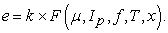(1)

Saxena and Seksena 10 showed that this expression can be separated into a product of two functions: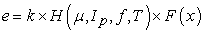(2)

and this implies that the expression (e1e2)/(e1 + e2) is independent of T, Ip, f and the coils’ design parameters: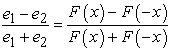(3)

Also, the denominator F(x) + F(-x) is a constant since an increase in F(x) equals a decrease in F(-x) 10. Hence, the quotient (e1 - e2)/(e1 + e2) is the key to a reliable LVDT demodulation.

Figures 3a to 3c illustrate the core’s position at the center position and at both extremes.

• Figure 3a. Centre position: e1 = e2
• Figure 3b. Left extreme: e1 = e1,min, e2 = e2,max
• Figure 3c. Right extreme: e1 = e1,max, e2 = e2,min

In Figure 3a, the core is at the neutral, midrange position and e1 = e2. In Figure 3b, the core is at the left end position and the e1 amplitude has a minimum (e1,min) and the e2 amplitude has a maximum (e2,max) and vice versa in Figure 3c. The e1 amplitude will change linearly from e1,min to e1,max when the core moves from -R to +R. Hence, the amplitude of e1 depends on x as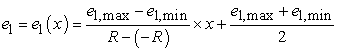(4)

and correspondingly for e2: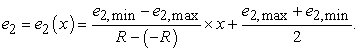(5)

Assuming that e2,max = e1,max = emax and e2,min = e1,min = emin, we have that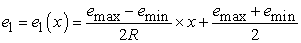(6)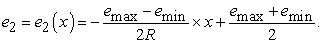(7)

It follows that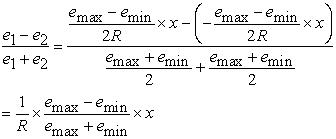(8)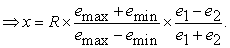(9)

In this work, e1 and e2 are detected by peak detectors and a 10-bit ADC. The output D from an n-bit ADC with input Uin is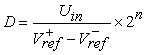(10)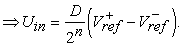(11)

In this work, the reference voltages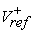and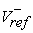will be tuned (by external potentiometers) to match exactly emax and emin, respectively. The consequence is that emax will correspond to an ADC output of 2n-1 and emin will produce an ADC output of 0. Hence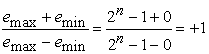(12)

and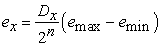(13)

Inserting (12) and (13) into (9) gives us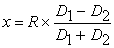(14)

Also, since D1 + D2 is a constant equal to 2n-1, we finally get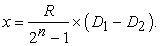(15)
2.1. Resolution

D1 - D2 ranges from -(2n-1) to 2n-1 when the core moves from -R to +R. This indicates a theoretical resolution of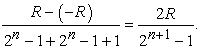(16)

The MHR250 LVDT used in this work has a nominal range of ±6.35 mm 33 and the microcontroller has an on-chip ADC with 10 bits resolution. The predicted resolution is therefore(17)
2.2. Uncertainty Analysis

In order to establish the expected uncertainty of x in (15), an uncertainty analysis is required 34. According to (15), x depends on D1, D2 and R (x = f(D1, D2, R)) and the uncertainty calculations depend on exact values of these parameters. In order to illustrate the order of magnitude of the uncertainty, we will assume some random numbers for D1 and D2 in the expected 10-bit range (D1 = 768 and D2 = 255). This will serve as an example of the size of the uncertainty and as a model for calculating the uncertainty in the general case. With D1 = 768, D2 = 255 and a (nominal) range of 6.35 mm, (15) predicts an x value of 3.184 mm.

The uncertainty of the ADC’s D values is ±0.5 (LSB) with a rectangular distribution and represents a standard uncertainty of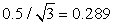34. Since only the nominal value of the range R is known (= 6.35 mm) it will be assumed to have a rectangular distribution with limits 6.350 ± 0.005 mm. This represents a standard uncertainty of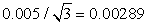mm.

The sensitivity coefficients are deduced by differentiating expression (15):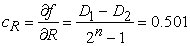(18)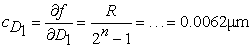(19)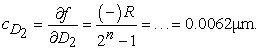(20)

Table 1 represents the uncertainty budget for the case where D1 = 768 and D2 = 255. An expansion coefficient of k = 2 has been used in order to get an uncertainty that represents a confidence interval of 95% 34.

Table 1 suggests an uncertainty in the estimated x position of 6 μm.

It could certainly be argued that the uncertainty analysis above is incomplete; the ADC’s D values depend on the reference voltagesandwith inherent uncertainties. However, we have assumed that they can be measured with a high enough certainty and accuracy not to contribute with any significant uncertainty to the overall uncertainty. (A 6½ digit 34401A Agilent DMM was used to measure the reference voltages.) Also, according to (15), x depends on n, but n is a constant with zero uncertainty.

### 3. Method and Material

3.1. Excitation

The heart of the signal conditioning electronics is a PIC16F1779 8-bit microcontroller in a 44 pin TQFP package from Microchip 35. This circuit hosts not only a CPU and ordinary microcontroller peripherals; it is an analog-digital hybrid with, for example, four OP amps. One of them is used for excitation of the primary LVDT coil and two are used to demodulate the secondary coils.

The excitation sinusoid is generated by filtering out the fundamental of a pwm generated square wave, using a second-order Butterworth filter in a traditional Sallen-Key circuit. This is illustrated in Figure 4.

Notice in Figure 4 that a) once the pwm is initiated, the sinusoid is self-maintained and requires no CPU attention and b) it only requires four external passive components (two resistors, two capacitors). According to the microcontroller’s datasheet, the OP amp has a driving capacity of 20 mA @ 4.5 volts output 35 indicating that the primary coil’s impedance must exceed 225 Ω. The LVDT was a MHR250 from TE Connectivity 33 and according to the datasheet it has an impedance of 147 Ω @ 2.5 kHz and 345 Ω @ 10 kHz. This would suggest that an excitation frequency of at least 6 kHz is required (pwm period ≤ 167 μs).

• Figure 4. Generation of excitation sinusoid
3.2. Demodulation

Since OP amps are available on-chip, demodulation was implemented as peak detector (PD) circuits. A PD follows a waveform until a maximum occurs and then holds the peak value until an even higher maximum occurs 36. PDs have found a wide range of applications in different disciplines. For example, in medicine they are used to monitor blood pressure and detection of hypertension 37, 38, 39, 40, 41 and detection of allergy levels 42. Figure 5 illustrates the traditional hardware implementation of a PD 43, 44.

• Figure 5. Classic peak detector

The OP amp will charge the capacitor through the diode as long as the input signal is rising and the Out voltage will follow the In signal exactly. When the In signal has reached a maximum and decreases, the capacitor will hold the maximum value since the diode is now reverse biased.

Figure 6 illustrates how the peak detectors are implemented in the PIC16F1779 microcontroller.

Each secondary coil is demodulated by a PD. The following details should be emphasized in Figure 6; a) the secondary coils’ sinusoids are bipolar and the reverse biased (germanium) diodes on the non-inverting inputs of the OP amps are necessary in order to protect the OP amp inputs from too negative voltages 35, b) each PD output is AD converted separately (AN12/AN16) and c) notice how each PD capacitor can be discharged by a dedicated I/O pin (RC3/RB4). During charging, this I/O pin is configured as digital input (high-Z) and the capacitor can be discharged by reconfiguring the pin in firmware to a digital output pin (set LOW).

• Figure 6. The LVDT signal conditioning proposal
3.3. Hardware/Experimental Setup

The entire signal conditioning circuitry is implemented on a 70x70 mm printed circuit board (pcb) and it has an 18-pin slot for a standard 2x20 row LCD display; the absolute core displacement is displayed on the LCD. Fig. 7 is a photo of the pcb. On the board are also three potentiometers; one is used to control the LCD contrast and the other two are used to set the ADC reference voltages to emax and emin, respectively (see Theory section). The LVDT is connected to the pcb via a simple 6-pin header connector.

Figure 8 is a photo of the experimental setup. The LVDT sensor house was fixed in position by a stand clamp and the core rod was attached to a micrometer translation board from Thorlabs which allowed the core position to be continuously shifted in precise micrometer steps (the micrometer screw has 10 μm notches).

3.4. Firmware

The firmware for the PIC16F1779 microcontroller was written in C using the standard XC8 C compiler available in Microchip’s MPLAB X® IDE (including Microchip’s Code Configurator) and downloaded to the controller (using an in-circuit debugger) via a 6-pin header connector on the pcb. The firmware is illustrated in Figure 9.

• Figure 9. Firmware flowchart

The main features of the software are the implementation of the peak detector circuits and the discharging of the capacitors by reversing the direction of two I/O pins and also that 50 x-values are averaged before the displacement is displayed on the LCD (see Analysis/Discussion section). The pwm period was set to 10 kHz suggesting a sinusoid period of 100 μs (which complies with the requirements deduced in the Theory section; T < 167 μs for the internal OP amp to be able to drive the primary coil).

### 4. Results

A digital oscilloscope was used to probe certain critical signals on the pcb to verify the performance. Figure 10 illustrates the sinusoid that was used to excite the primary coil.

Period and amplitude agree exactly with expectations. The quality of the sinusoid was determined by exporting the waveform data to MATLAB and performing an FFT analysis in order to calculate the THD; a THD of 3.3% was found and is very close to the generally accepted limit of 2-3% 1. (Figure 10 reveals a minor, periodic glitch in the sinusoid (this glitch is discussed in the Analysis/Discussion section later).

In order to establish the correct reference voltage levels for the microcontroller’s ADC, it is imperative to measure the secondary coils’ outputs at both extremes. This is illustrated in Figure 11 and Figure 12.

Notice in Figure 11 and Figure 12 the impact of the protection diodes in Figure 6; when the signal is < -0.3 volts, the sinusoid is cut off. From Figure 11 and Figure 12 it was concluded that the assumption that e1,max = e2,max and e1,min = e2,min is justified and from the peak values, the reference voltages could be determined to= 0.812 V and= 1.772 V.

By gradually shifting the core rod from -6.350 mm to +6.350 mm (by turning the micrometer screw on the translation board), the LCD reading was registered. Due to noise, there was some minor flickering in the reading and the maximum and minimum display values were registered and used to assign error bars to the plot. The display reading as a function of the displacement of the core is plotted in Figure 13. Figure 14 and Figure 15 represent magnifications of certain areas in Figure 13 where the core was translated in smaller steps. A linear fit line is plotted in the same graph for reference.

• Figure 10. Sinusoid with THD = 3.3 %
• Figure 11. Secondary coils, left extreme
• Figure 12. Secondary coils, right extreme
• Figure 13. Calibration results
• Figure 14. Core position ∈[-0.45..+0.45 mm]
• Figure 15. Core position ∈[-0.050..+0.050 mm]
• Figure 16. Core position ∈[-0.065..-0.045 mm]
• Figure 17. Deviation scatter plot

Finally, to determine the true resolution, the micrometer screw on the translation board was set to “inter-notch” positions, i.e. right between two 10 μm notches (representing 5 μm steps). This is illustrated in Figure 16.

Figure 17 represents a deviation scatter plot, i.e. the difference between the linear fit line data and the displayed data produced in this work.

### 5. Analysis/Discussion

The suggested solution for LVDT signal conditioning was satisfactory confirmed. The overall linearity of the signal response in Figure 13 was slightly better than 1%.

The initial tests revealed the presence of noise in the output signal of the order of 18 μm (= std of Gaussian distribution). This was remedied by averaging a number of readings (50) before displaying the displacement information.

This averaging will have an adverse impact on the overall bandwidth but, NB, it has a favorable impact on the resolution; due to the presence of random noise the resolution can be improved beyond the theoretical limit of 6.2 μm. Averaging samples with random noise facilitates a means to interpolate between the inherent integer steps of an ADC 45. Due to the limited resolution of the micrometer translation board (10 μm notches) it was not possible to unequivocally determine the exact resolution experimentally, but figure 16 suggests a 1 μm resolution.

The excitation sinusoid in Fig. 10 has an unexpected periodic glitch of unknown origin. A likely explanation is that it stems from the original pwm signal; the sharp edges of the pwm signal are transferred to the output sinusoid by capacitive or inductive cross-talk. It could be argued that if that is the case, the glitches should appear exactly at the zero-crossings of the sinusoid, but that could be explained by a phase-shift in the sinusoid caused by the RC network of the Sallen-Key filter. Anyway, the glitches do not seem to have any influence on the performance and no further efforts were conducted to analyze them or eliminate them; due to the low-pass characteristics of the transfer function between primary and secondary coils, the glitches are not transferred to the secondary coils output. (Even if they were, the PDs would ignore them.)

In retrospect, the design has a slight flaw; the design depends on the fact that the ADC’s reference voltages can be tuned to the emax and emin voltages exactly. On this pcb, single-turn potentiometers were used which made the tuning of the reference voltages precarious; multiple-turn trimming potentiometers should be used to facilitate precise tuning of the reference voltages.

The deviation scatter plot in Figure 17 reveals a slightly higher error mid-range between the end points and “0” and an order of magnitude smaller errors close to the “0” position. This is (most likely) due to an inherent non-linearity in the LVDT itself, but it should be noted that this could easily be compensated for in firmware in the proposed design, suggesting an overall linearity limit much better that 1%.

### 6. Conclusions

A seminal signal conditioning solution for LVDTs has been presented. Due to the low-cost components used (a PIC16F1779 is \$1.92), the solution offers a cost-efficient alternative to commercial signal conditioning chips without any degradation of performance parameters. The total cost of the semiconductors on the pcb is of the order of \$3, (excluding the LCD). The pcb itself was ordered online from a Chinese pcb factory at a cost of \$1 (five pcbs for \$5). Only an analog/digital hybrid chip, four diodes, a couple of resistor-capacitor pairs and two potentiometers are used to produce a resolution of the order of single micrometers (after averaging), a typical uncertainty of 6 μm and a linearity < 1%.

### Acknowledgements

The author would like to thank eddylab GmbH (https://www.eddylab.com/) for providing the cross-sectional sketch of an LVDT in Figure 2.

### ReferencesThis work is licensed under a Creative Commons Attribution 4.0 International License. To view a copy of this license, visit http://creativecommons.org/licenses/by/4.0/